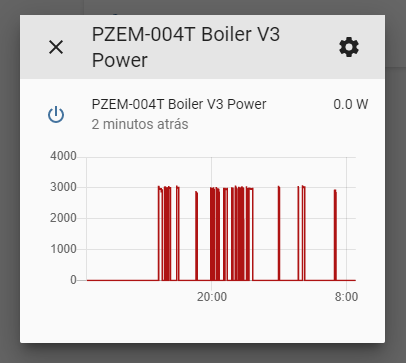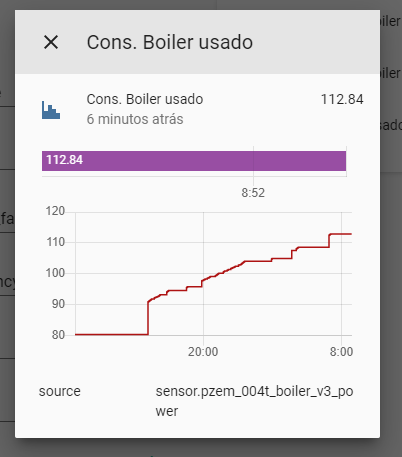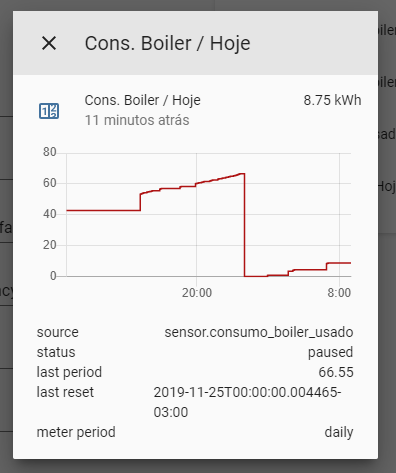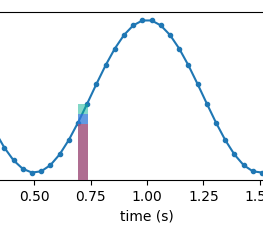# Integration sensor wrong / inaccurate

Hi,
I’ve purchased a PZEM-004T and integrated it into Home Assistant with ESPHome. It works just fine and reports the devices value as Watts in realtime (fetches every 3 seconds), just like shown below:To calculate the used kWh, I saw that using an integration sensor would be the right thing to do, so I put into the configuration.yaml:

``````  - platform: integration
source: sensor.pzem_004t_boiler_v3_power
name: energy_spent
unit_prefix: k
round: 2
``````

But when I analyse the spent energy, it reports wrong values. Sometimes if the “Boiler” uses 3kW for about 15 min the usage suddenly goes +8kWh or similar, which is highly inaccurate.(See as it suddenly jumps to 90kWh and does some other jumps)

This, on the other hand, makes the daily graph inaccurate too:If I look at the graphs manually I would say that it used at most 3-4kWh, but not 8.7kWh (which would mean it would have ran 3kW for almost 3h, which, according to the graphs, seems like this isn’t the case).

If anyone can tell me what is going on or how to ‘fix’ this, it would be awesome.

Thank you!

1 Like

Does anyone have this problem?

1 Like

Well mathematically speaking, if your boiler is at 3000 w every 3 seconds for 15 minutes, the summation of the integration will be 2kw/15minutes which is 8kw/hr. So it’s not wrong if that’s what your saying.

And which would be the right way to configure the sensor to report the right values? It should be 3kW for one hour, in theory, not 3kW in 15 min…

It’s a summation of the area under the curve. So if it was only on for 15 minutes in an hour the result would be 2kW. If it was on the whole hour it would be 8kW. Are you sure this is the calculation that you want?

I’m lost as to what 3000 W (not w) every 3 seconds is, does that mean (and I assume) that it averaged 3kW over the 3 seconds ?
(kW is power, kWh is energy - I know you know this I’m just stating it for the record) power is instantaneous and energy is defined by an added dimension of time
So lets assume 3kW in 1 second (actually as I said it’s instantaneous, but Ws (Watt seconds) is viable)
3kW per sec is 180kW per min or 10,800kW per hour or 10.8MWh

What is this boiler ? (I bought a fairly big boiler at 45kWh)

Edit: multiple edits to confirm dimensions and the arithmetic (no maths required apart from the dimensions)

Nah I’ve got something wrong here !
It must be averaging Wh (Watt Hours)

What type of boiler is it ?
If it’s gas then presumably you have a flowmeter attached and the ‘meter’ is measuring volume at an assumed pressure and calorific value ???
If it’s electric then it’s measuring Current and an “Assumed” voltage (or measured voltage) ? And are these RMS values ?
As a heat load - power factor will be close to unity

I’ll assume that Valentino is European and therfore is at 220v ac
nominal heater loads would be circa 3kW but I have seen 40kW industrial heaters (but few above 8kW in water systems).
3kW equates to about 13.54 amps, Thats a pretty big contactor but it’s possible (our hob has a 40amp breaker and switch, The US would need even bigger as they are 110v)

I think you’re getting lost over the equation, which is a trapezoidal integration which is the sum of the area under a curve. Which is why i’m asking if that’s what he really wants. The equation is

summation of ((previous value + this value) * time) / 2. I.E getting the area under a single value.

In his case…

area = ((3000+3000) * 3) / 2.

Then ingratiating it and converting to his time format…

integration = area / ( 1000 * 3600 ), 1000 for kW and 3600 for an hour.

which is about 0.0025 per 3 seconds gives us (0.0025 * 1200) = 3kW/hr. So my calc’s in my previous post are wrong as I was using 15 minutes. I’ll edit that out. Either way, I verified that the calculation is using that.

So… @Valentino_Stillhardt ignore my wrong calculations before. You should only be getting 8k beyond 2 hours of data.

Hmm !
Lost over the equation, no I don’t think so.
By definition energy is integrated over time (I don’t think

is required)
So the sensor reading is 3k ‘something’ (I now think Wh) and is always 3k (look at his sensor) which means you only have to add the times up (of device ‘on’) you have the energy used in that period.
What happens if he turns the switch on for only 2 secs ? I reckon he’ll still get 3000 thingumies
So seconds on * 3,000Wh /3600 = kWh correction : I NOW think it will provide an arithmetic mean over the sample time of the sensor, but the calc is the same

Edit : so if its on continuously for 1 hour (3600 secs) then the result is 3kW, hey the rating of the device or if its only on for 1800 seconds (half an hour) only 1.5kWh (you can sumate over a month and get kWh for that month too)

it’s not actually integrating, its using Riemann sums to approximate because we don’t have an equationThanks for all the anwers, I really appreciate it.

I think I’ve explained something wrong. Those 3 seconds are the “scan_interval” of the sensor. Every 3 seconds it reads the power usage. The boiler uses 3000W (3kW) when on, but it is on only for a couple of minutes a day and suddenly the reported kWh gets ridiculously high, but I don’t know why.

It should report 1kWh used for 1h of continuous 3kW use, but it seems to jump much higher than this.

Sorry Valentino but that is wrong - if I use 3kW in 1 second (ie instantaneous usage) then by definition I have used 3 kWh in 1 hour (assuming continuous usage)

Is it electric what is the current rating ?

Would that mean if I used 3kW for a couple of seconds or minutes it’ll stay at 3kWh until it reaches one hour? Or am I getting this wrong?

No, if (say) you switch it on for 10 seconds then you’ll probably get 4 readings of 3 kW (as you say updated every 3 seconds) but 10 seconds on at 3kw equals
3kW * 10s / 3600 seconds = 8.3 Wh or 3/10ths of sod all (not very much)

Yes, this makes sense…

But I’m getting 8kWh, even if the boiler was on (on total) for only an hour maybe.

I now put the integration method instead of the default “trapezoidal” to “left”, and now it seems to count upwarts instead of just jumping up (I think).

Yeah, I don’t use any of the stats package so I can’t help with that.
Do you want kWh per day, per hour, per what ?
But what I’d do (clunky and sub optimal etc) would be either to count the updates it’s on in the above period multiply by 3 secs * 3 kW and divide by 3600
Or better still just count seconds on (per you period) * 3kW and divide by 3600
They will both give you kWh but the second is more accurate

Maybe you need to wait for someone who knows the stats packagee

You should NEVER get more than 3kWh in any 1 hour, use the above to check your output

I wanted the used kWh per day. It works, resets daily and shows the usage from the previous day, but too high. But I could be wrong, of course.

I used something similar: To test I created a template sensor which goes to “on” when the usage is above 2500W (when the boiler is heating), else “off”. Then I used a history statistic sensor which tells me how much time the sensor was “on”. Based on the time it was “on” I made a sensor in which I used: `3 * hours_on` and it tells me how much kWh it used. This is accurate, but not 100%, because the boiler doesn’t use 3000W all the time, sometimes it uses 2900 and such, but it should suffice to test if both come to a similar value. I’ll report back!

You still haven’t said what type of boiler you are running or how the sensor works

As I said the stats are not an area I can comment on but it might help if you change the name of the thread to “stats package help needed for sensor” or similar just to encourage the right people to attend it

You’d probably want right over left. Left takes the old state, right takes the new state. Trapezoidal takes an average of the old and the new.

EDIT: Actually it’s really just up to you. Here’s a visual difference. Red = left, blue = trap, green = right. It describes the area under the curve that is being compounded.1 Like

It’s a PZEM-004T, which is a 100A CT Clamp sensor which you connect on the ESP8266 or ESP32 to measure energy usage, voltage, PF, etc.

I hope I did understand you right…